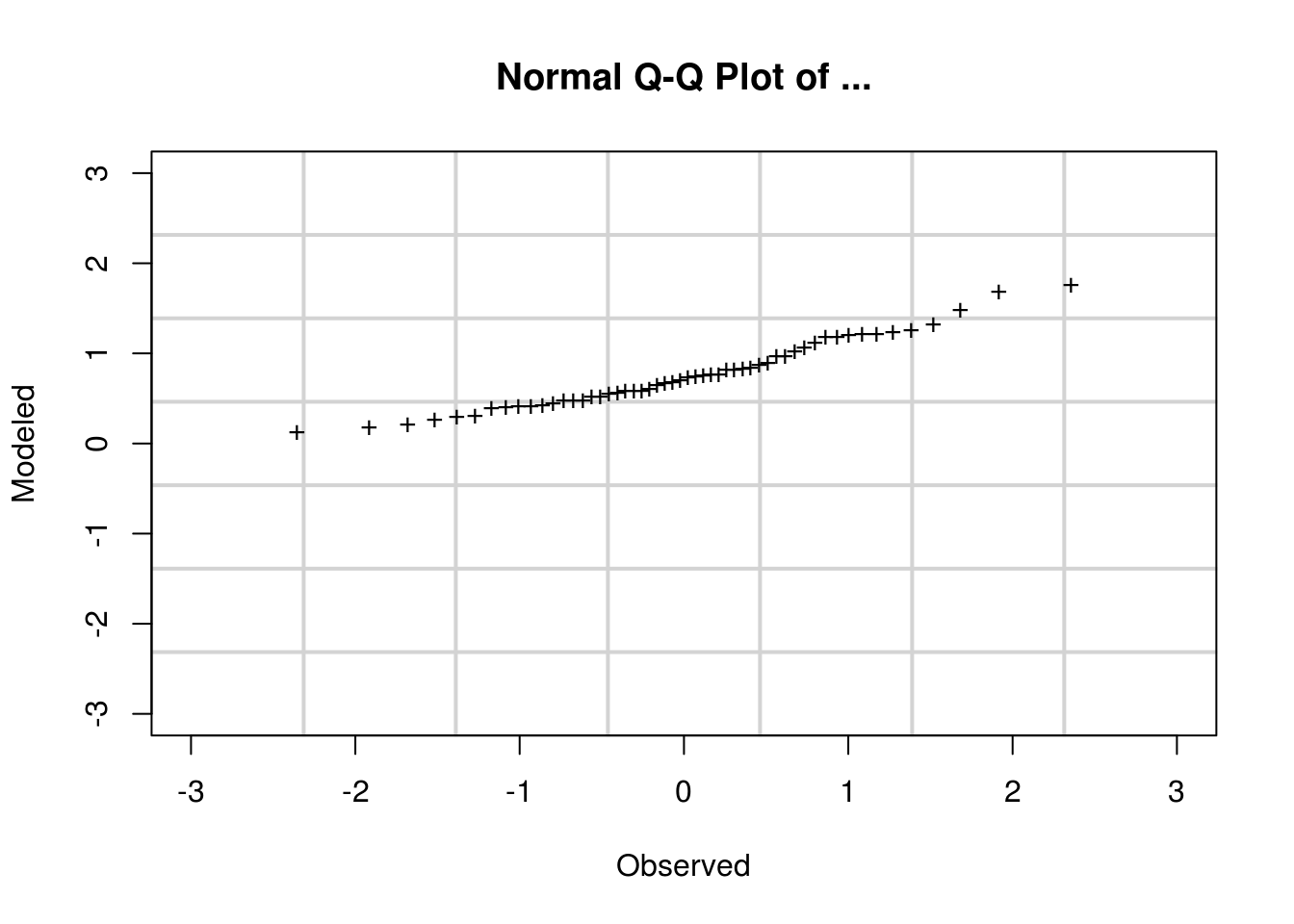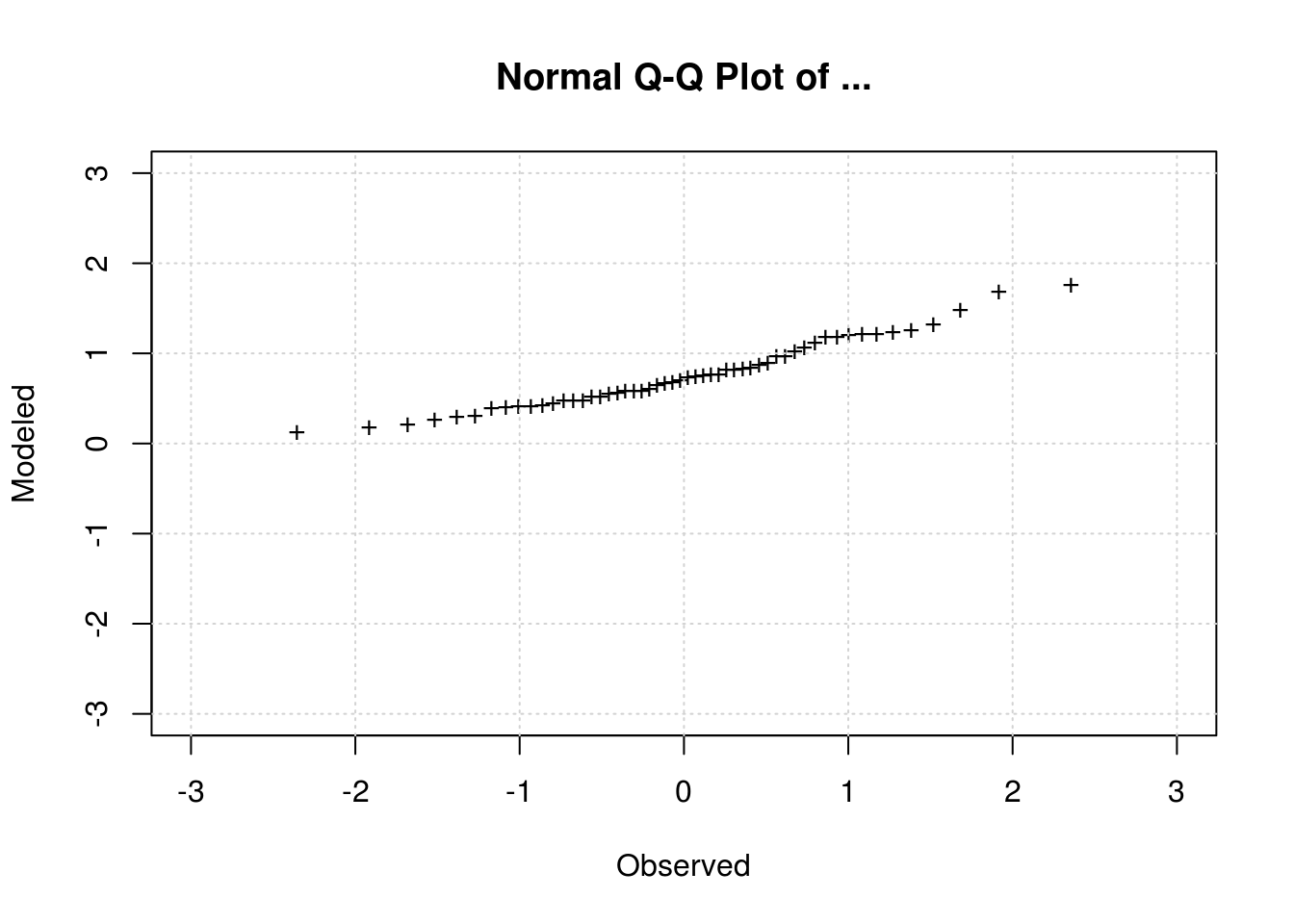## 12 September 2014

### How to make QQ plot in R

Dear friends,

For those of you that are still having some problems making chart in R, here’s an example for QQ plot. I made it steps.

1. Read data

Use your own data.

QQTest <- read.table(file="QQTest.dat",sep=",",head=TRUE)

2. Make a QQplot

qqnorm(QQTest$vals)3. Annotate the plot qqnorm(QQTest$vals,
main="Normal Q-Q Plot of ...",
xlab="Observed",
ylab="Modeled")4. Make the same x and y limit

qqnorm(QQTest$vals, main = "Normal Q-Q Plot of ...", xlab = "Observed", ylab = "Modeled", xlim = c(-3, 3), ylim = c(-3, 3))5. Change to + symbol qqnorm(QQTest$vals,
main = "Normal Q-Q Plot of ...",
xlab = "Observed",
ylab = "Modeled",
xlim = c(-3, 3),
ylim = c(-3, 3),
pch = "+")Add the QQ line

qqline(QQTest$vals, col = "blue", lwd = 2, lty = 2) Or make it red qqline(QQTest$vals, col = "red", lwd = 2, lty = 2)

Or make it not dotted

qqline(QQTest$vals, col = "blue", lwd = 2, lty = 1) Or make it thinner qqline(QQTest$vals, col = "blue", lwd = 1, lty = 1)

6. Add the grid

qqnorm(QQTest$vals, main = "Normal Q-Q Plot of ...", xlab = "Observed", ylab = "Modeled", xlim = c(-3, 3), ylim = c(-3, 3), pch = "+", panel.first = grid(7, lty = 1, lwd = 2))Not good right? Try this one qqnorm(QQTest$vals,
main = "Normal Q-Q Plot of ...",
xlab = "Observed",
ylab = "Modeled",
xlim = c(-3, 3),
ylim = c(-3, 3),
pch = "+")
abline(v=(seq(-3, 3, by=1)), col="lightgray", lty="dotted")
abline(h=(seq(-3, 3, by=1)), col="lightgray", lty="dotted")7. Make two columns plot: side by side

First delete your old plot using plot.new() then change the layout

plot.new()
par(mfrow=c(2, 1))

Make the left plot

qqnorm(QQTest$vals, main = "Upper Plot -> Normal Q-Q Plot of ...", xlab = "Observed", ylab = "Modeled", xlim = c(-3, 3), ylim = c(-3, 3), pch = "+") abline(v=(seq(-3, 3, by=1)), col="lightgray", lty="dotted") abline(h=(seq(-3, 3, by=1)), col="lightgray", lty="dotted") Then make the right plot qqnorm(QQTest$vals,
main = "Right Plot -> Normal Q-Q Plot of ...",
xlab = "Observed",
ylab = "Modeled",
xlim = c(-3, 3),
ylim = c(-3, 3),
pch = "+")
abline(v=(seq(-3, 3, by=1)), col="lightgray", lty="dotted")
abline(h=(seq(-3, 3, by=1)), col="lightgray", lty="dotted")

Or make it two rows: upper and lower

plot.new()
par(mfrow=c(1, 2))

Make the upper plot

qqnorm(QQTest$vals, main = "Upper Plot -> Normal Q-Q Plot of ...", xlab = "Observed", ylab = "Modeled", xlim = c(-3, 3), ylim = c(-3, 3), pch = "+") abline(v=(seq(-3, 3, by=1)), col="lightgray", lty="dotted") abline(h=(seq(-3, 3, by=1)), col="lightgray", lty="dotted") Then make the lower plot qqnorm(QQTest$vals,
main = "Lower Plot -> Normal Q-Q Plot of ...",
xlab = "Observed",
ylab = "Modeled",
xlim = c(-3, 3),
ylim = c(-3, 3),
pch = "+")
abline(v=(seq(-3, 3, by=1)), col="lightgray", lty="dotted")
abline(h=(seq(-3, 3, by=1)), col="lightgray", lty="dotted")

There you go. You make more tweaks it based on your needs. You can also use a specific package with specific style like: lattice or ggplot2. Make sure you read the documentation first, as each package may have different commands, but the basic principle is the same.

Good luck.

@dasaptaerwin